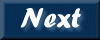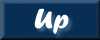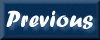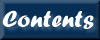Next: 5. Programs Up: XRONOS User's Guide Previous: 3. Walk through   Contents

Subsections

# 4. Input Files

The input file format is FITS using the BINTABLE extension in either binned data format or event list format. To specify multiple files a Filelist is recommended.

# 4.1 File format

The FITS input file format that XRONOS read is described in the "The proposed Timing FITS file format for High Energy Astrophysics data", 1993, by Angelini, L. , W. Pence and A.F. Tennant (OGIP memo OGIP/93-003 http://heasarc.gsfc.nasa.gov/docs/journal/timing3.html ). The FITS input file are BINTABLE extension with two possible layouts one for binned data and another for event list. For both formats a column TIME is required in addition to timing header keywords. The TIME column is not required if data are equispaced and the gap marked. The require timing header keywords are the following:

```    MJDREF  =                        / MJD for reference time
TSTART  =                        / start time
TSTOP   =                        / stop stop
TIMEZERO=                        / zero time used to calculate the
n-time event or the n-time
TIMESYS =                        / the system use to the define time
TIMEUNIT=                        / Unit for TSTART, TSTOP, TIMEZERO
valid also for the two keywords
rapresentation
TIMEDEL =                        / Integration time
```

TIMESYS records the time frame system used in the file. The IAU FITS Working Group in 1997 adopted this keyowrd as part of the FITS date definition encopassing the millenium reccomended a number of values (see http://www.cv.nrao.edu/fits/documents/standards/year2000.txt ) not included in the Angelini et al. (1994). Prior that decision the following values for TIMESYS were used :

```     1- an arbitrary UT time, given as decimal years or as 'YYYY MM DD hh:mm:ss'
(e.g. TIMESYS = '1980.0'  or TIMESYS = '1980 1 1 00:00:00';
2- MDJ, JD, or TJD which define the various Julian day representations,
mainly to accomodate older mission;
3- 'scc' (for spacecraft clock used in the ROSAT data).
Note TIMESYS is not a required keyword.
```

MJDREF is the reference time given as a MJD, using the following convention MJD=JD-2400000.5. Time written in the TIME column and in the timing header keywords are offset from this value. MJDREF is not required and if missing the values in the TIME column are treated as absolute. This quantity can be specified in two keywords, MJDREFI and MJDREFF for integer and fraction, when necessary to retain precision.

TSTART, and TSTOP give the start and the stop of the data included in file in units specified by the TIMEUNIT keyword. These quantities can be specified in two keywords, TSTARTI and TSTARTF (TSTOPI and TSTOPF) for integer and fraction, when necessary to retain precision. These keywords are required. If MJDREF is present they are offset from MDJREF, otherwsie should have values comparable (written in the same time system) to those in the TIME column. TSTART and TSTOP should include also any offset stored in the TIMEZERO keyword.

TIMEZERO is an offset used to calculate the time for each bin or event in the table when the TIME column is not present. This quantity can be specified in two keywords, TIMEZERI and TIMEZERF for integer and fraction, when necessary to retain precision. Note that if the TIME column is present and TIMEZERO is not zero this value is added to the values in the time colum and the result value should be always included in the interval defined by the TSTART and TSTOP. The value of TIMEZERO should be written in the same time system of TSTART and TSTOP and with units defined in TIMEUNIT.

TIMEUNIT gives the time unit used for the TSTART, TSTOP, and TIMEZERO keywords. It is a required keyword. TIMEUNIT is used by the software to make the value of the keywords compatible with the units of the 'TIME' column. The recommended units code follows the IAU convention, i.e. 's' for second and 'd' for day, and is described in OGIP/93-001 document (George and Angelini 1993). If 's' is specified it is assumed that all the time header keywords are in units of seconds (TSTART, TSTOP and TIMEZERO).

TIMEDEL gives the integration time of the data. NOTE that TIMEDEL can be applied to binned data or to event data (for example CCD data). The units are defined in TIMEUNIT.

The TIME column contains either the tagged time for each event or the center of the bin. The time can be expressed as residual time from TIMEZERO (that in turn is an offset from MJDREF if defined) or as full time since MJDREF or an user defined time system. The TIME column values for binned data always refer to the center of the bin, unless the keyword TIMEPIXR specify otherwise. The units are stored in the TUNITn keyword with recommended unit abbreviations of 's' for seconds and 'd' for days.

TIMEPIXR gives the position of time stamp value within a bin. The tipical values are 0 for the beginning of the bin, 0.5 for the middle of the bin and 1 for the end of the bin. If TIMEPIXR is absent, the default value is 0.5 (This keyword was not present in the OGIP/93-003)

## 4.1.1 Event data

An Event file has only one required column, 'TIME', which gives the recorded time for each event. Other column as PHA can be included when the instrument for example allows data to be resolved in energy. The important key is that each row (n) contains single-value parameters for the event n. The Event table must be accompany by a GTI extension to allow to determine the exposure in each bin. A tipical event table suitable as xronos input file can have the following header:

```     MJDREF  = 49718                / MJD for reference file
TIMESYS = '1995 1 1 00:00:00 ' / The time system is MJD
TIMEUNIT= 'd       '           / unit for TSTARTI/F and TSTOPI/F
TSTART  =  800                 / observation start time
TSTOP   =  801                 / observationstop time
TIMEZERO= 0.0                  / zerotime to calculate t(n) event or bin
TTYPE1  = 'TIME    '           / time of measurement
TFORM1  = '1D      '           / data format of the field: DOUBLE PRECISION
TUNIT1  = 'd       '           / physical unit of field
```

Note: The EXTNAME or HDUCLAS1 keyword must have the value "EVENT" for xronos to recognize it as an events file.

## 4.1.2 Bin data

The binned data table requires the columns, RATE (ERROR), COUNTS which contain the intensity of the source detected in the integrated bin. Additional column can be present as TIME or FRACEXP. The FRACEXP contians the fraction exposure, compared with the integration time stored in the header keywords TIMEDEL, with value ranging from 0 to 1 (full exposure). The TIME column is data are not equispaced or gap are not marked.

An example header for binned data containing rate or counts are listed below:

```     MJDREF  = 49718                / MJD for reference file
TIMESYS = '1995 1 1 00:00:00 ' / The time system is MJD
TIMEUNIT= 'd       '           / unit for TSTARTI/F and TSTOPI/F
TSTART  =  800                 / observation start time
TSTOP   =  801                 / observationstop time
TIMEZERO= 0.0                  / zerotime to calculate t(n) event or bin
TIMEDEL = 1                    / integration time
TTYPE1  = 'TIME    '           / time of measurement
TFORM1  = '1D      '           / data format of the field: DOUBLE PRECISION
TUNIT1  = 'd       '           / physical unit of field
TTYPE2  = 'RATE'               / data from detector channel
TFORM2  = '1E      '           / data format of the field: 4-byte REAL
TUNIT2  = 'count/s'            / physical unit of field
TTYPE3  = 'ERROR   '           / error in intensity
TFORM3  = '1E      '           / data format of the field: 4-byte REAL
TUNIT3  = 'count/s'            / physical unit of field
TTYPE4  = 'FRACEXP '           / error in intensity
TFORM4  = '1E      '           / data format of the field: 4-byte REAL
```

```     MJDREF  = 49718                / MJD for reference file
TIMESYS = '1995 1 1 00:00:00 ' / The time system is MJD
TIMEUNIT= 'd       '           / unit for TSTARTI/F and TSTOPI/F
TSTART  =  800                 / observation start time
TSTOP   =  801                 / observationstop time
TIMEZERO= 0.0                  / zerotime to calculate t(n) event or bin
TIMEDEL = 1                    / integration time
TTYPE1  = 'TIME    '           / time of measurement
TFORM1  = '1D      '           / data format of the field: DOUBLE PRECISION
TUNIT1  = 'd       '           / physical unit of field
TTYPE2  = 'COUNTS  '           / data from detector channel
TFORM2  = '1E      '           / data format of the field: 4-byte REAL
TUNIT2  = 'count   '           / physical unit of field
TTYPE3  = 'FRACEXP '           / error in intensity
TFORM3  = '1E      '           / data format of the field: 4-byte REAL
```

An example header for equispaced binned data with no time column is listed below:

```     MJDREF  = 49718                / MJD for reference file
TIMESYS = '1995 1 1 00:00:00 ' / The time system is MJD
TIMEUNIT= 'd       '           / unit for TSTARTI/F and TSTOPI/F
TSTART  =  800                 / observation start time
TSTOP   =  801                 / observationstop time
TIMEZERO= 800                  / zerotime to calculate t(n) event or bin
TIMEDEL = 1                    / integration time
TTYPE1  = 'RATE'               / data from detector channel
TFORM1  = '1E      '           / data format of the field: 4-byte REAL
TUNIT1  = 'count/s'            / physical unit of field
TTYPE2  = 'ERROR   '           / error in intensity
TFORM2  = '1E      '           / data format of the field: 4-byte REAL
TUNIT2  = 'count/s'            / physical unit of field
TTYPE3  = 'FRACEXP '           / error in intensity
TFORM3  = '1E      '           / data format of the field: 4-byte REAL
```

# 4.2 Filelist

To input multiple files for each time series, a file containing the list of files is needed (Filelist). The Filelist is input in the program as @Filelist. The format of this file list is ascii and contains one filename+options per line. Files from different times series are separated by '///' mark. Below is an example of the Filelist containing 2 files for 3 different times series.

```    file1_ser1
file2_ser1
///
file1_ser2
file2_ser2
///
file1_ser3
file2_ser3
```

# 4.3 Input File Options

The Input File Options (up to 10) can be specified for each file in the same input string. They consist of 2 characters followed by a numerical constant (up to 8 character long). There are two groups of options. The first allows data selection within a FITS extension. The available options within this group are :

• frN= start reading input file from row number N (First Row)
• lrN= stop reading input file at row number N (Last Row)
• vxN= use column number N as x-axis (i.e. time axis, default: TIME)
• vyN= use column number N as y-axis (default: COUNT or RATE)
• vsN= use column number N as error for y-axis (default: ERROR)
• veN= use column number N as exposure (default: FRACEXP) If the input file is an event list, exposure is by default calculated using the GTI extension. In this case, N=0 turns off the usage of the GTI extension for the exposure calculation, and N0 specifies the GTI extension to use.
• feN= select data (either binned or events) from channel number N (First Energy). For event list the default column channel name searched is PHA. The option vcN allows the choice of a channel column name different from PHA (es. PI).
• leN= select data (either binned or events) to channel number N (Last Energy). Event list channel selection is identical to feN
• vcN= use column number N for channel selection (valid only for event lists)
• rtN= use extension N of the FITS file to read the data. The first extension is N=1 (the primary array is irrelevant). To specify the extension the following also can be used: filename[N] or filename+N.
• of = The MJDREF keyword is not used. The time is calculated using the TIME column and the TIMEZERO keyword.

The second group of options performs algebraic operations on individual input files. They are applied in the same order in which are specified. For event files they are applied after the data are binned. The available options within this group are:

• stX= Shift all Times in input file by X days
• ssX= Shift all times in input file by X Seconds
• muX= multiply data and errors by X (MUltiply)
• mdX= multiply data by X (Multiply Data)
• meX= multiply errors by X (Multiply Errors)
• maX= as muX but exposure is divided by X (used for dead time correction)
• diX= divide data and errors by X (DIvide)
• ddX= divide data by X (Divide Data)
• deX= divide errors by X (Divide Errors)
• daX= as diX but exposure is multiplied by X
• saX= subtract data and errors with X (Subtract All)
• sdX= subtract data with X (Subtract Data)
• seX= subtract errors with X (Subtract Errors)
• qaX= add to data the square of data multiplied by X and add to errors the product of data and error multiplied by X. (This is used to multiply data (Y) and errors (SY) by a linear interpolation constant: Y'= Y(A+BY)= AY+BYAYA/AA= YA+CYAYA with C=B/AA , SY'= SY(A+BY)= SYA+BYASYA/AA= SYA+CYASYA. This can be done by using options: muA qaC (where A and C are those used above)
• qdX= as above but for data only
• qeX= as above but for errors only

Below is an example of the Filelist containing 2 files for 3 different times series where the different options are applied to the input files for different time series.

```    file1_ser1 aa4           add to data and error 4
file2_ser1 aa4            "      "        "    "
///
file1_ser2 rt2 aa2       read 2nd extension; add to data and error 2
file2_ser2 rt2 aa2        "    "     "           "    "        "   "
///
file1_ser3 rt2 vy4 vs5   read 2nd extension; use column 4 and 5 for Y-axis and Error
file2_ser3 rt2 vy4 vs5    "    "    "         "    "    "     "  "    "          "
```Next: 5. Programs Up: XRONOS User's Guide Previous: 3. Walk through   Contents